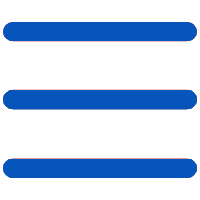﻿ 期刊影响因子（JCR）-图书馆门户网站### 期刊影响因子（JCR）

Impact Factor影响因子

Impact Factor影响因子（IF）：23.605

IF=(C2+C3)/(A2+A3)

A3=1994年出版的文献数=1054（篇）

C2=1995年出版的文献在1996年被引用的次数=24189（次）

C3=1994年出版的文献在1996年被引用的次数=25170（次）

IF =(24189+25170)/(1037+1054)= 23.605（次/篇）